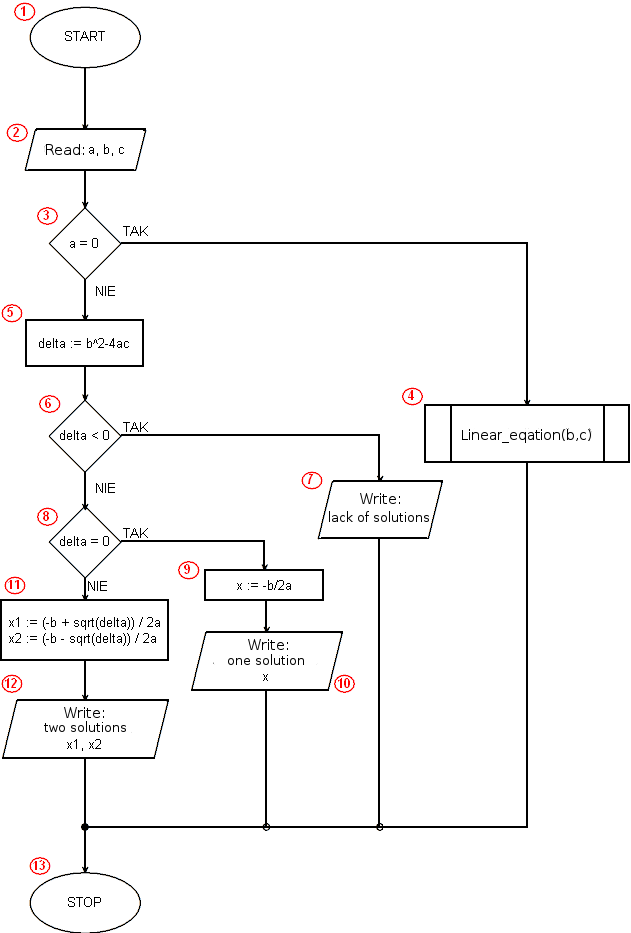# Implementation of the algorithm solving a quadratic equation in JavaScript<!DOCTYPE html>
<html>
<body>
<script>

function linear(a,b) {
if (a==0)
if (b==0)
else
else {
var x = -b / a;
}
}

var a=prompt("a:", "");
var b=prompt("b:", "");
var c=prompt("c:", "");
if (a == 0)
liniowe(b,c);
else {
var delta = b*b-4*a*c;
if (delta < 0)
else
if (delta == 0) {
var x = -b / (2 * a);
alert("One solution x = " + x);
}
else {
var x1 = (-b + Math.sqrt(delta)) / (2 * a);
var x2 = (-b - Math.sqrt(delta)) / (2 * a);
alert("x1 = " + x1 + "    x2 = " + x2);
}
}

</script>
</body>
</html>

 Program line Description Algorithm block 1 In the 1st line of the program, we inform the browser that our document was written in HTML (more precisely, it is a header specific to HTML 5). Line 2 contains the tag that begins the HTML code. The 3rd line starts the section of our document, containing the elements that are to appear on the page. In our example, the section contains only a script written in JavaScript that implements the algorithm for solving a quadratic equation. The , i . -